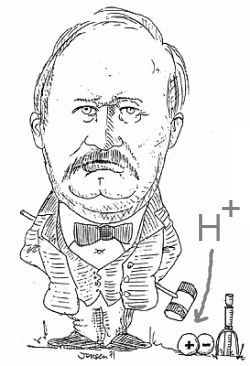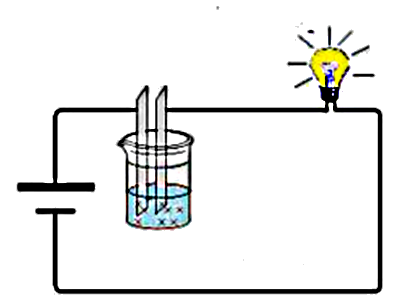Search:

# Acids and bases

## Arrhenius definitionSvante Arrhenius (1859-927)

## Definitions

An acid is a substance which liberates in water the hydrogen ion $H^+$ A base is a substance which liberates in water the hydroxide ion OH^-

## Examples

Chlorhydric acid $HCl$: $HCl$ $_\longrightarrow^{water}$ $H^+$ $+$ $Cl^-$ Sulfuric acid $H_2SO_4$: $H_2SO_4$ $_\longrightarrow^{water}$ $H^+$ $+$ $HSO_4^-$ Phosphoric acid $H_3PO_4$: $H_3PO_4$ $_\longrightarrow^{water}$ $H^+$ $+$ $HPO_4^{2-}$ The base sodium hydroxide $NaOH$: $NaOH$ $_\longrightarrow^{water}$ $Na^+$ $+$ $OH^-$ The base calcium hydroxide $Ca(OH)_2$: $Ca(OH)_2$ $_\longrightarrow^{water}$ $Ca^{2+}$ $+$ $2OH^-$

## Discussion

### The term "substance" (= neutral chemical species)

Colour indicators show whether a solution is acidic, basic or neutral, for instance bromothymol:in 1) acid 2) neutral 3) basic medium Let's test this indicator with three salt solutions containing each two species of ions:

 Ammonium chloride Sodium chloride Sodium hydroxide $NH_4^+$ $Na^+$ $Na^+$ $Cl^-$ $Cl^-$ $OH^-$ yellow green blue

It appears clearly that it is not the $Cl^-$ ion which is acidic, but rather the ammonium ion $NH_4^+$. Likewise it is not the $Na^+$ ion which is basic, but rather the $OH^-$ ion

The definition of acids and bases should not be limited to substances, but ions must also be included

### The solvent "water"

Ions produced by the dissociation of an acid in water carry the electric current:Let's test the influence of the solvent on that dissociation:

 Benzene Alcohol WaterIt appears that alcohol allows also the dissociation of acids to ions!

The definition of acids and bases should not be limited to water as solvent.

### The "$H^+$" ion

The hydrogen atom $H$ has one proton making its nucleus and one electron making its electron cloud. If we take away this electron, we produce the hydrogen ion $H^+$ which is in fact nothing else than a mere proton. Because of its very small diameter and its positive charge, all surrounding molecules must be very strongly attracted by this ion, especially if they are polarized. ( the attraction between two charges of opposite signs is inversely proportional to the square of their distance !)!

The hydrogen ion $H^+$ cannot exist alone in an aqueous solution !

## Conclusion

Therefore it was necessary to extend the simple Arrhenius definition to other chemical species , other solvents and to drop the notion of a free $H^+$ ion in solution. This important step was taken by Johannes Nikolas Brønstedt.